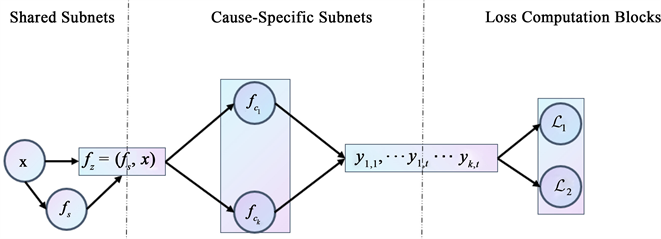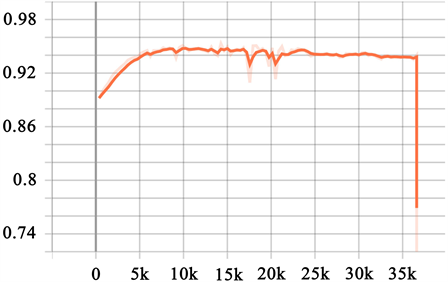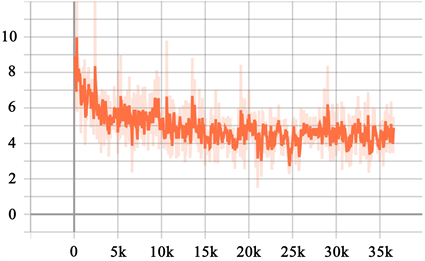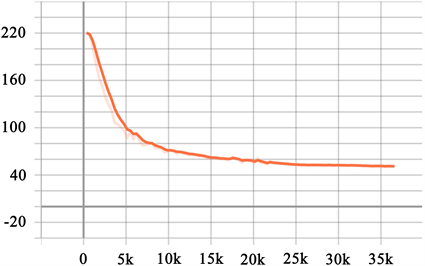#### 期刊菜单

Application Research on Practical Survival Problems Based on Deep Learning
DOI: 10.12677/SA.2022.114091, PDF, HTML, XML, 下载: 38  浏览: 77

Abstract: Disease is a major problem that has plagued all human health and even life since ancient times. Survival analysis is a method that can simulate the survival of patients, and can understand the relationship between interested events and covariates, such as the relationship between the death time of a cancer patient and his age, gender and other covariates. In recent years, the application of survival analysis has become more and more extensive. It has also achieved great development not only in hospitals, but also in other industries such as e-commerce, advertising, telecommunications and financial services. Through survival analysis, these companies can better understand when customers buy products, when they will lose customers, and when they will default on loans. This paper uses a deep learning based survival analysis model, DeepHit model, to process the real data set and compare it with other models. It is found that DeepHit model has a good effect.

1. 背景

2. 数据及模型介绍

2.1. 数据来源

SUPPORT数据集(表1)来自一项以预测9105名重症住院患者在180天内的生存率的研究。在9105名患者中，6201 (68.1%)名患者被随访直至死亡，生存时间中位数为58天，平均生存时间478.45天。SUPPORT数据含括了年龄、性别、种族等30个代表患者信息的协变量。

2.2. 数据预处理

2.3. 模型介绍

DeepHit模型通过训练神经网络学习估计事件和时间的联合分布。生存模型由一个共享网络和K个特定原因的子网络组成，并使用softmax层作为最终的输出层输出模型学习的K个竞争事件的联合分布和每个原因的边缘分布如图1所示。

$\begin{array}{l}{\alpha }_{1}={W}_{11}\ast {X}_{1}+{W}_{12}\ast {X}_{2}+{W}_{13}\ast {X}_{3}+{b}_{1}\\ {\alpha }_{2}={W}_{21}\ast {X}_{1}+{W}_{22}\ast {X}_{2}+{W}_{23}\ast {X}_{3}+{b}_{2}\\ {\alpha }_{3}={W}_{31}\ast {X}_{1}+{W}_{32}\ast {X}_{2}+{W}_{33}\ast {X}_{3}+{b}_{3}\end{array}$Figure 1. Model structure

K个特定原因子网络层将 $z=\left({f}_{s}\left(x\right),x\right)$ 作为输入，学习协变量共有表示的向量 ${f}_{s}\left(x\right)$ 和潜在因素，输出特定原因K的第一次命中的时间的概率，这些输出的汇总是在首次命中事件和时间上的联合概率分布，病因特异性子网并行学习每个病因的首次命中时间的边缘分布。

$\begin{array}{l}{F}_{{k}^{*}}\left({t}^{*}|{x}^{*}\right)=P\left(s\le {t}^{*},k={k}^{*}|x={x}^{*}\right)\\ \text{}=\underset{{s}^{*}=0}{\overset{{t}^{*}}{\sum }}P\left(s={s}^{*},k={k}^{*}|x={x}^{*}\right)\end{array}$

3. 实证分析Figure 2. CIFFigure 3. Train lossFigure 4. Val loss

4. 结论

DeepHit是一种基于深度学习的生存分析方法，本位通过使用DeepHit模型处理SUPPORT真实数据集，模型通过使用深度学习方法，利用共享子网络层和特定因子层直接学习了生存时间和生存事件的联合分布，并对其进行估计并推导出CIF的估计值。

  Fine, J.P. and Gray, R.J. (1999) A Proportional Hazards Model for the Subdistribution of a Competing Risk. Journal of the American Statistical Association, 94, 496-509. https://doi.org/10.1080/01621459.1999.10474144  Lee, C., Zame, W.R., Yoon, J., et al. (2018) DeepHit: A Deep Learning Approach to Survival Analysis with Competing Risks. Proceedings of the AAAI Conference on Artificial Intelligence, 32. https://doi.org/10.1609/aaai.v32i1.11842  Nagpal, C., Li, X. and Dubrawski, A. (2021) Deep Survival Machines: Fully Parametric Survival Regression and Representation Learning for Censored Data with Competing Risks. IEEE Journal of Biomedical and Health Informatics, 25, 3163-3175. https://doi.org/10.1109/JBHI.2021.3052441  Katzman, J.L., Shaham, U., Cloninger, A., et al. (2018) DeepSurv: Personalized Treatment Recommender System Using a Cox Proportional Hazards Deep Neural Network. BMC Medical Research Methodology, 18, Article No. 24. https://doi.org/10.1186/s12874-018-0482-1